# 1. 背景介绍

## 0x1：单信源二元信号统计检测的基本模型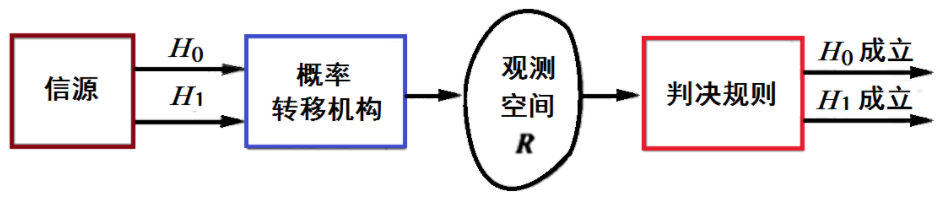• 信源：检测的信号发生源
• 概率转移机构：它是在信源输出其中一个为真的基础上，把噪声干扰背景中的假设Hj(j=0,1​)为真的信号以一定概率关系映射到观测空间。
• 如果没有噪声干扰，信源输出的某一种确知信号将映射到观测空间中的某一确定点
• 但在噪声干扰的情况下，它将以一定的概率映射到整个观测空间
• 观测空间：观测空间 R 是在信源输出不同信号状态下，在噪声干扰背景中，由概率转移机构所生成的全部可能观测量的集合
• 判决规则：观测量落入观测空间后，就可以用来推断哪一个假设成立是合理的，即判决信号属于哪种状态。为此需要建立一个判决规则，以便使观测空间中的每一个点对应着一个相应的假设Hi(i＝0,1)。判决结果就是选择假设 H0 成立，还是选择假设 H1 成立。例如最大后验估计就是一种判决规则。所谓判决规则，本质上是对观测空间R的划分问题。

### 3、观测空间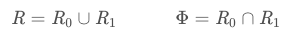### 4、判决规则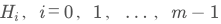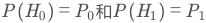，且 P0 + P1 = 1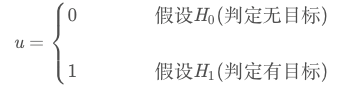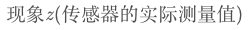• H0 为真、判决 u=1 为第一类错误：虚警
• H1 为真、判决 u=0 为第二类错误：漏检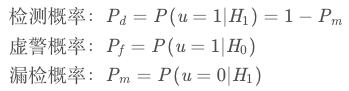• R0：如果数据点 z 位于 R0 区，则认为假设 H0 成立而做出决策 u=0；
• R1：如果数据点 z 位于 R1 区，则认为假设 H1 成立而做出决策 u=1；

## 0x2：单信源二元信号检测的数学描述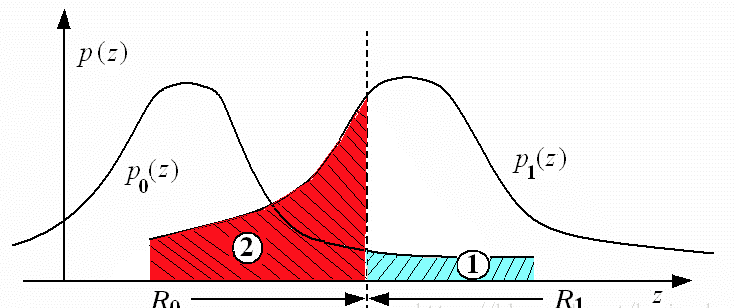# 2. 多源检测融合的系统架构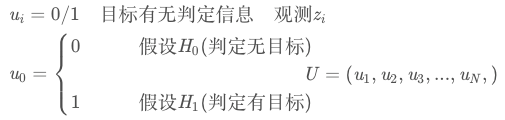# 3. 多源信号融合的准则

## 0x2：最大后验概率融合检测准则

• 若 P(H1|u) > P(H0|u)，则选择 H1
• 反之选择 H0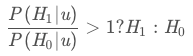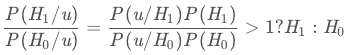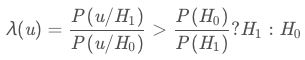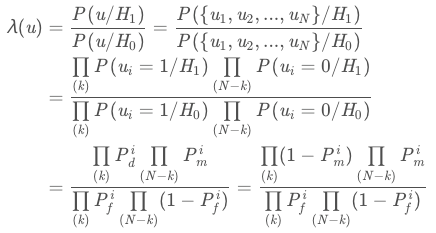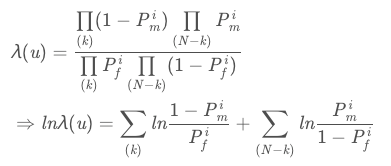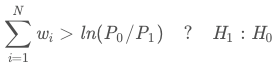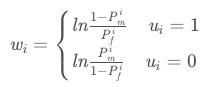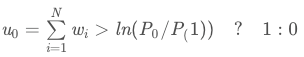• u0=1表示有目标
• 否则视为无目标

## 0x3：贝叶斯融合检测基本准则

### 1、准则形式化描述

Cij 为假设 Hi 成立时做出 uj 决策的代价，平均总代价为：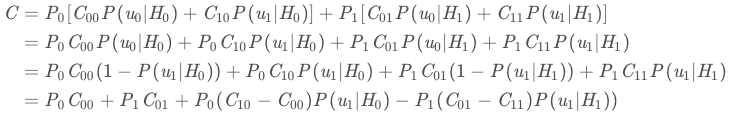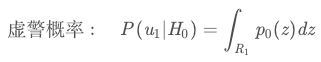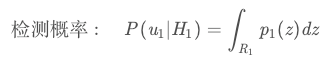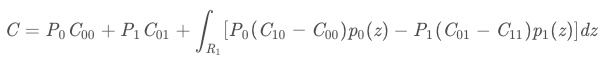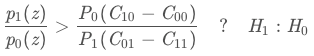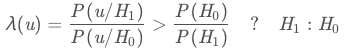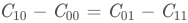### 2、贝叶斯融合检测准则讨论

• C10 - C00 大：即虚警引起的损失大，门限应取大一些使虚警出现的可能性小一点；反之亦然。
• C01 - C11 大：即漏检引起的损失大，门限应取小一些使漏检出现的可能性小一点；反之亦然。

## 0x4：最小误差概率准则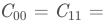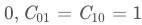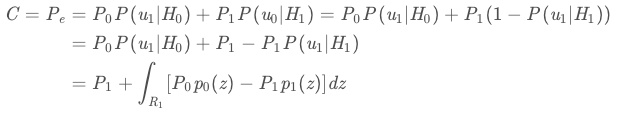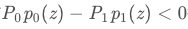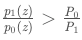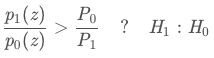# 4. 基于贝叶斯融合理论的多源信号融合算法

## 0x1：多源信号贝叶斯融合公式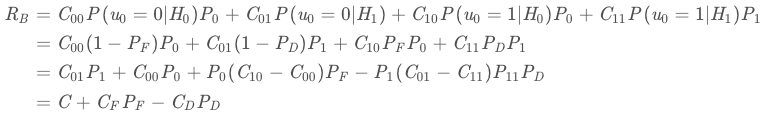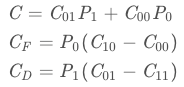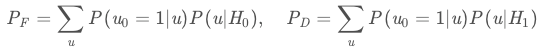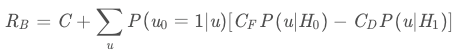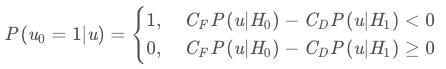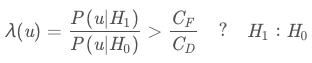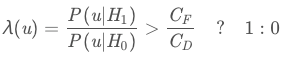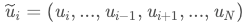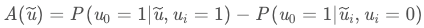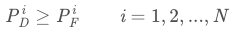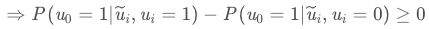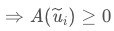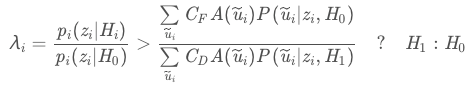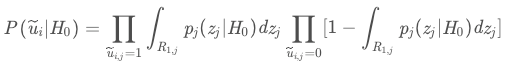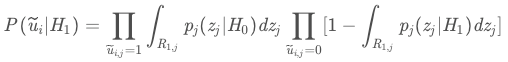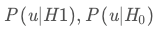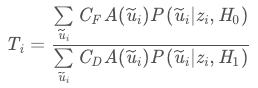## 0x2：求解贝叶斯融合检测准则最佳判决门限的迭代算法

### 1、第一步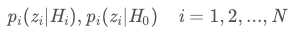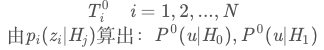### 2、第二步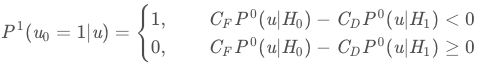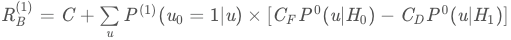### 3、第三步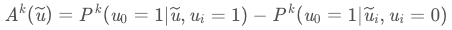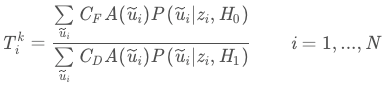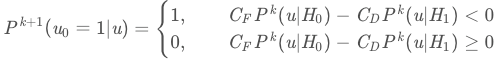### 4、第四步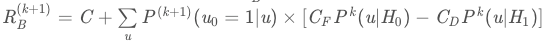# 5. 仿真实验

## 0x1：实验背景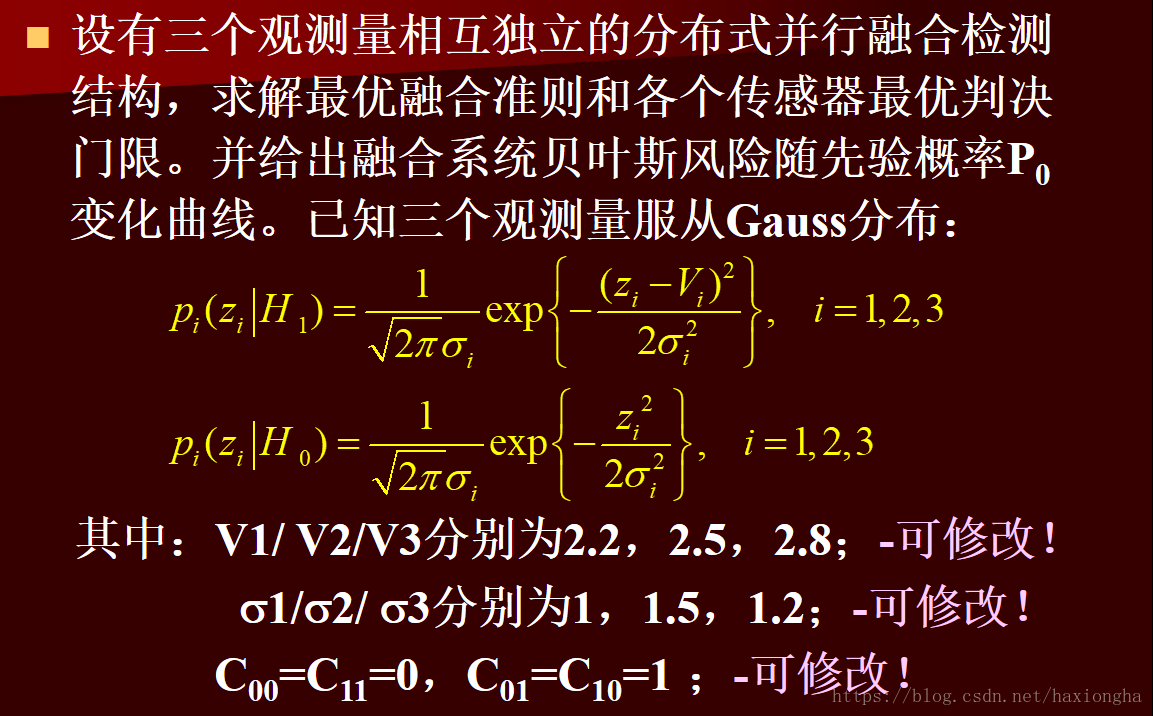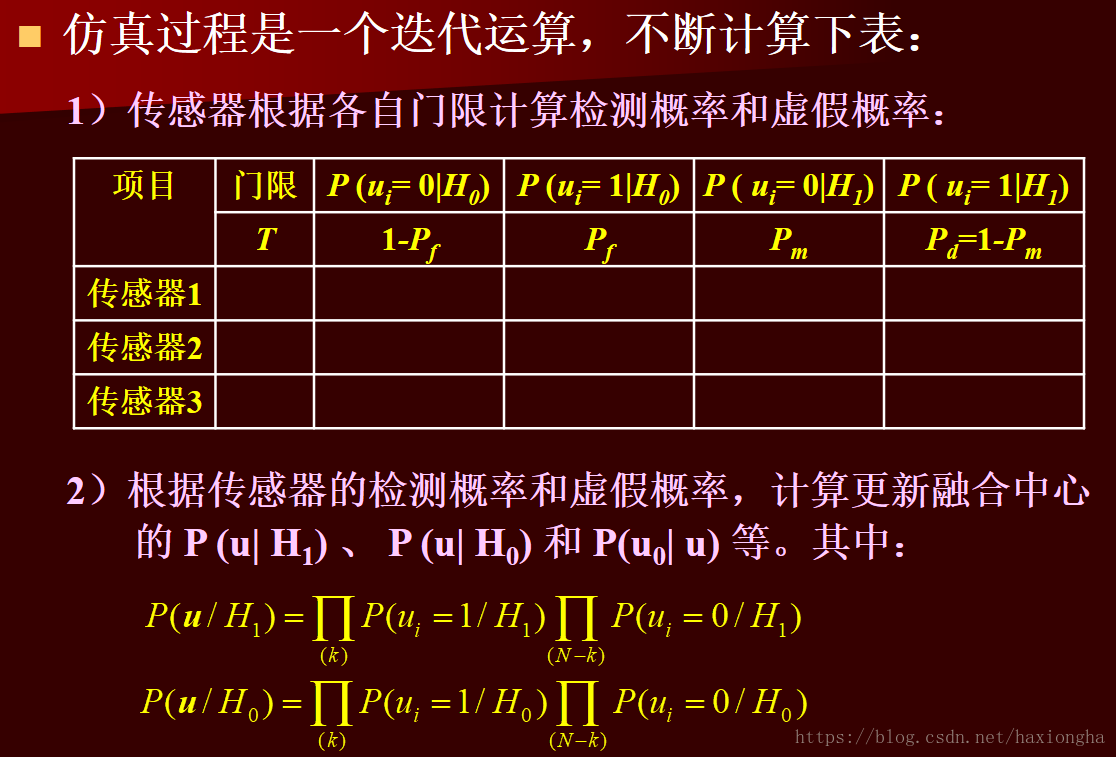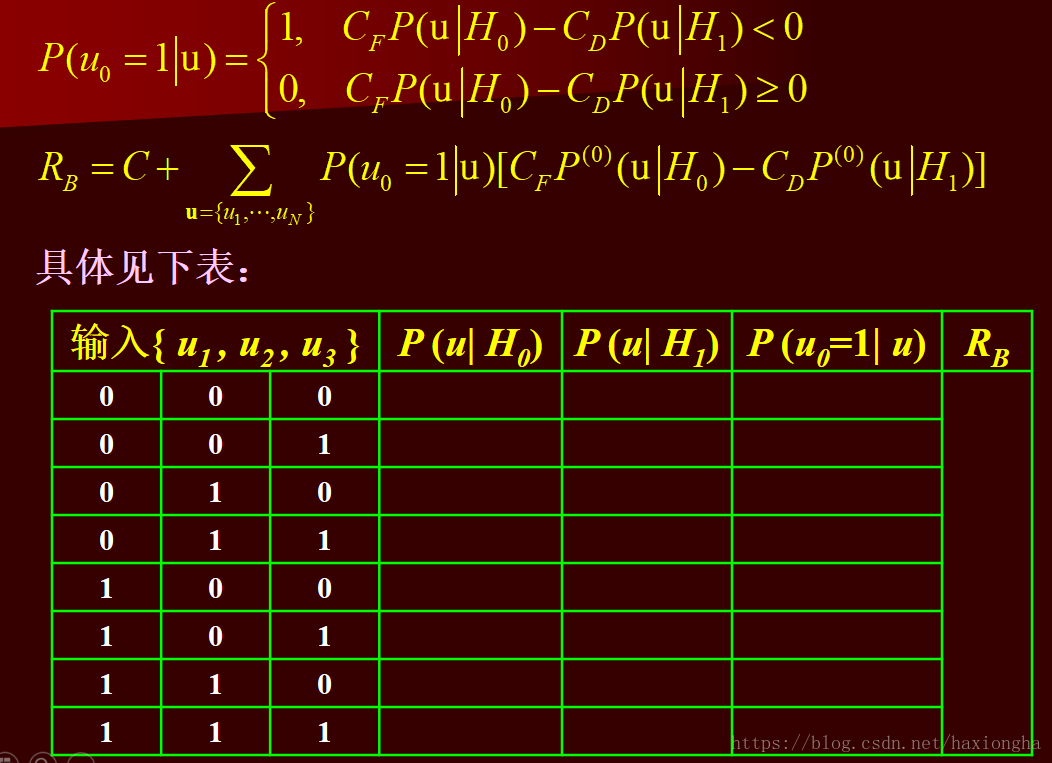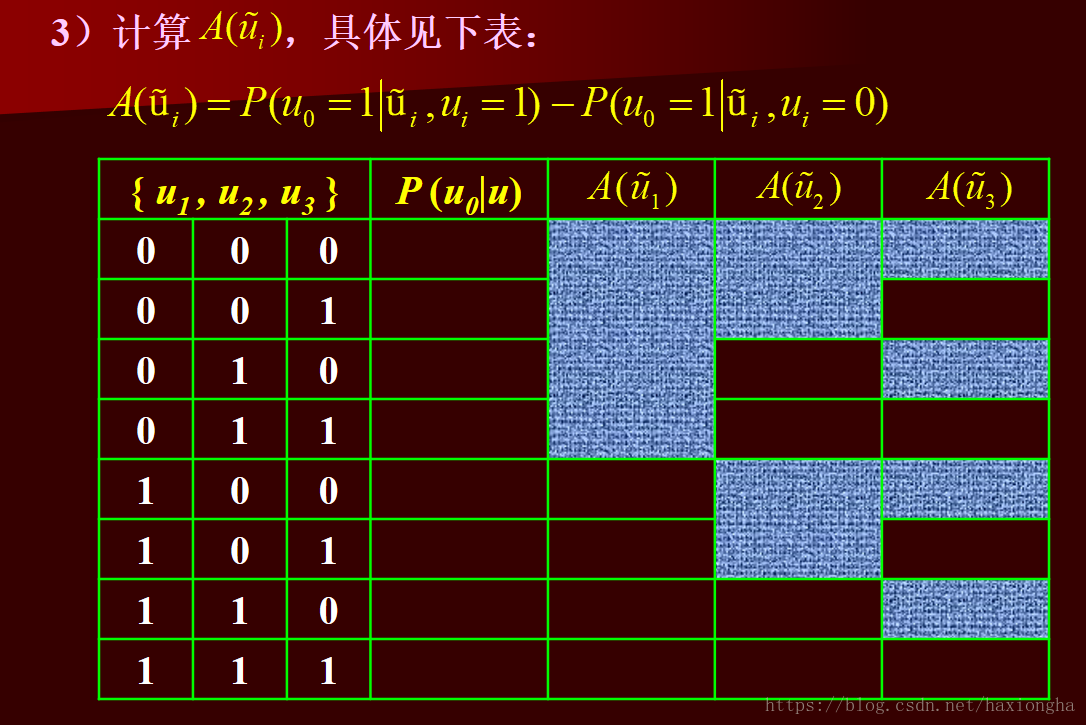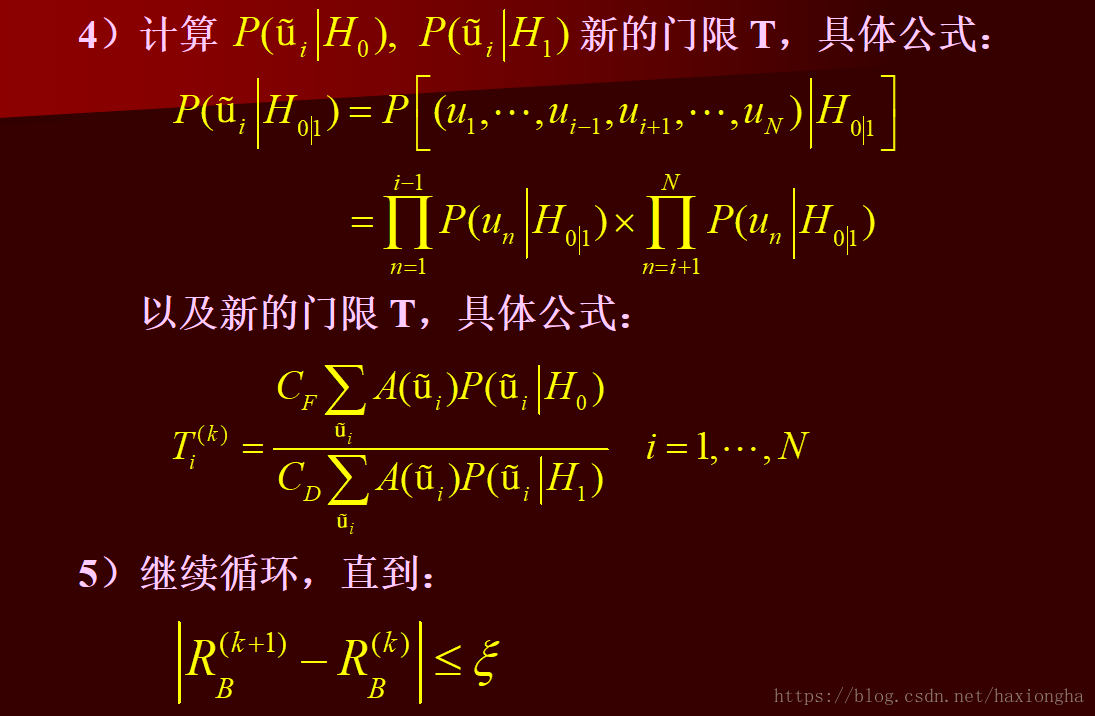clc;
j = 100;
V1 = 2.2;
V2 = 2.5;
V3 = 2.8;
delta1 = 1;
delta2 = 1.5;
delta3 = 1.2;

%初始化三个传感器的门限值
gama = zeros(j,3);

%代价初始化
C00 = 0;
C11 = 0;
C01 = 1;
C10 = 1;

%传感器1概率初始化
pf1 = zeros(j,1);
pm1 = zeros(j,1);
pd1 = zeros(j,1);
p001 = zeros(j,1);

%传感器2概率初始化
pf2 = zeros(j,1);
pm2 = zeros(j,1);
pd2 = zeros(j,1);
p002 = zeros(j,1);

%传感器3概率初始化
pf3 = zeros(j,1);
pm3 = zeros(j,1);
pd3 = zeros(j,1);
p003 = zeros(j,1);

Rb = zeros(j,1);

%先验概率初始化
P0=1/j:(1/j):1;
P1 = 1 - P0;

C = C01*P1 + C00*P0;
CF = P0*(C10-C00);
CD = P1*(C01-C11);
t = (CF./CD);

%初始判决门限，因为初始情况下，门限公式后面的无法计算

gama(1,1) = t(1);
gama(1,2) = t(1);
gama(1,3) = t(1);

for i = 1:j
%根据门限计算各个传感器的四类概率
p001(i) = normcdf(gama(i,1),0,delta1);
pm1(i) = normcdf(gama(i,1),V1,delta1);
pd1(i) =1 -  pm1(i);
pf1(i) = 1 - p001(i);

p002(i) = normcdf(gama(i,2),0,delta2);
pm2(i) = normcdf(gama(i,2),V2,delta2);
pd2(i) =1 -  pm2(i);
pf2(i) = 1 - p002(i);

p003(i) = normcdf(gama(i,3),0,delta3);
pm3(i) = normcdf(gama(i,3),V3,delta3);
pd3(i) =1 -  pm3(i);
pf3(i) = 1 - p003(i);

%根据传感器的检测概率和虚假概率，计算融合中心融合准则
Pu000_H1 = pm1(i)*pm2(i)*pm3(i);
Pu000_H0 = p001(i)*p002(i)*p003(i);

Pu001_H1 = pm1(i)*pm2(i)*pd3(i);
Pu001_H0 = p001(i)*p002(i)*pf3(i);

Pu010_H1 = pm1(i)*pd2(i)*pm3(i);
Pu010_H0 = p001(i)*pf2(i)*p003(i);

Pu011_H1 = pm1(i)*pd2(i)*pd3(i);
Pu011_H0 = p001(i)*pf2(i)*pf3(i);

Pu100_H1 = pd1(i)*pm2(i)*pm3(i);
Pu100_H0 = pf1(i)*p002(i)*p003(i);

Pu101_H1 = pd1(i)*pm2(i)*pd3(i);
Pu101_H0 = pf1(i)*p002(i)*pf3(i);

Pu110_H1 = pd1(i)*pd2(i)*pm3(i);
Pu110_H0 = pf1(i)*pf2(i)*p003(i);

Pu111_H1 = pd1(i)*pd2(i)*pd3(i);
Pu111_H0 = pf1(i)*pf2(i)*pf3(i);

Pu0_1_u000 = (Pu000_H1/Pu000_H0) > t(i);
Pu0_1_u001 = (Pu001_H1/Pu001_H0) > t(i);
Pu0_1_u010 = (Pu010_H1/Pu010_H0) > t(i);
Pu0_1_u011 = (Pu011_H1/Pu011_H0) > t(i);
Pu0_1_u100 = (Pu100_H1/Pu100_H0) > t(i);
Pu0_1_u101 = (Pu101_H1/Pu101_H0) > t(i);
Pu0_1_u110 = (Pu110_H1/Pu110_H0) > t(i);
Pu0_1_u111 = (Pu111_H1/Pu111_H0) > t(i);

%计算融合系统的贝叶斯风险

Rb(i) = C(i) + Pu0_1_u000*(CF(i)*Pu000_H0 - CD(i)*Pu000_H1) + ...
Pu0_1_u001*(CF(i)*Pu001_H0 - CD(i)*Pu001_H1)+...
Pu0_1_u010*(CF(i)*Pu010_H0 - CD(i)*Pu010_H1)+...
Pu0_1_u011*(CF(i)*Pu011_H0 - CD(i)*Pu011_H1)+...
Pu0_1_u100*(CF(i)*Pu100_H0 - CD(i)*Pu100_H1)+...
Pu0_1_u101*(CF(i)*Pu101_H0 - CD(i)*Pu101_H1)+...
Pu0_1_u110*(CF(i)*Pu110_H0 - CD(i)*Pu110_H1)+...
Pu0_1_u111*(CF(i)*Pu111_H0 - CD(i)*Pu111_H1);

%计算A(u)，确定某一个传感器的值后组合两外两个传感器的值
%确定u1
Au1_00 = Pu0_1_u100 - Pu0_1_u000;
Au1_01 = Pu0_1_u101 - Pu0_1_u001;
Au1_10 = Pu0_1_u110 - Pu0_1_u010;
Au1_11 = Pu0_1_u111 - Pu0_1_u011;

%确定u2
Au2_00 = Pu0_1_u010 - Pu0_1_u000;
Au2_01 = Pu0_1_u011 - Pu0_1_u001;
Au2_10 = Pu0_1_u110 - Pu0_1_u100;
Au2_11 = Pu0_1_u111 - Pu0_1_u101;

%确定u3
Au3_00 = Pu0_1_u001 - Pu0_1_u000;
Au3_01 = Pu0_1_u011 - Pu0_1_u010;
Au3_10 = Pu0_1_u101 - Pu0_1_u100;
Au3_11 = Pu0_1_u111 - Pu0_1_u110;

%计算后验概率
Pu1_00_H0 = p002(i)*p003(i);
Pu1_01_H0 = p002(i)*pf3(i);
Pu1_10_H0 = pf2(i)*p003(i);
Pu1_11_H0 = pf2(i)*pf3(i);

Pu2_00_H0 = p001(i)*p003(i);
Pu2_01_H0 = p001(i)*pf3(i);
Pu2_10_H0 = pf1(i)*p003(i);
Pu2_11_H0 = pf1(i)*pf3(i);

Pu3_00_H0 = p001(i)*p002(i);
Pu3_01_H0 = p001(i)*pf2(i);
Pu3_10_H0 = pf1(i)*p002(i);
Pu3_11_H0 = pf1(i)*pf2(i);

Pu1_00_H1 = pm2(i)*pm3(i);
Pu1_01_H1 = pm2(i)*pd3(i);
Pu1_10_H1 = pd2(i)*pm3(i);
Pu1_11_H1 = pm2(i)*pm3(i);

Pu2_00_H1 = pm1(i)*pm3(i);
Pu2_01_H1 = pm1(i)*pd3(i);
Pu2_10_H1 = pd1(i)*pm3(i);
Pu2_11_H1 = pm1(i)*pm3(i);

Pu3_00_H1 = pm1(i)*pm2(i);
Pu3_01_H1 = pm1(i)*pd2(i);
Pu3_10_H1 = pd1(i)*pm2(i);
Pu3_11_H1 = pm1(i)*pm2(i);

%计算三种传感器的融合判决门限

T1 =(CF(i)*(Au1_00*Pu1_00_H0 + Au1_01*Pu1_01_H0 + Au1_10*Pu1_10_H0 + Au1_11*Pu1_11_H0))/...
(CD(i)*(Au1_00*Pu1_00_H1 + Au1_01*Pu1_01_H1 + Au1_10*Pu1_10_H1 + Au1_11*Pu1_11_H1));

T2 =(CF(i)*(Au2_00*Pu2_00_H0 + Au2_01*Pu2_01_H0 + Au2_10*Pu2_10_H0 + Au2_11*Pu2_11_H0))/...
(CD(i)*(Au2_00*Pu2_00_H1 + Au2_01*Pu2_01_H1 + Au2_10*Pu2_10_H1 + Au2_11*Pu2_11_H1));

T3 =(CF(i)*(Au3_00*Pu3_00_H0 + Au3_01*Pu3_01_H0 + Au3_10*Pu3_10_H0 + Au3_11*Pu3_11_H0))/...
(CD(i)*(Au3_00*Pu3_00_H1 + Au3_01*Pu3_01_H1 + Au3_10*Pu3_10_H1 + Au3_11*Pu3_11_H1));

gama(i+1,1) = log(T1)/V1 + V1/2;
gama(i+1,2) = log(T2)/V2 + V2/2;
gama(i+1,3) = log(T3)/V3 + V3/2;

end
%传感器1贝叶斯风险
R1 = (C00*p001+C10*pf1).*P0' + (C01*pm1+C11*pd1).*P1';
%传感器2贝叶斯风险
R2 = (C00*p002+C10*pf2).*P0' + (C01*pm2+C11*pd2).*P1';
%传感器3贝叶斯风险
R3 = (C00*p003+C10*pf3).*P0' + (C01*pm3+C11*pd3).*P1';

plot(P0,R1,'b',P0,R2,'g',P0,R3,'black',P0,Rb,'r');
title('贝叶斯风险曲线图');
xlabel('p0');
ylabel('贝叶斯风险值');
legend('传感器1贝叶斯风险曲线','传感器2贝叶斯风险曲线','传感器3贝叶斯风险曲线','融合系统贝叶斯风险曲线');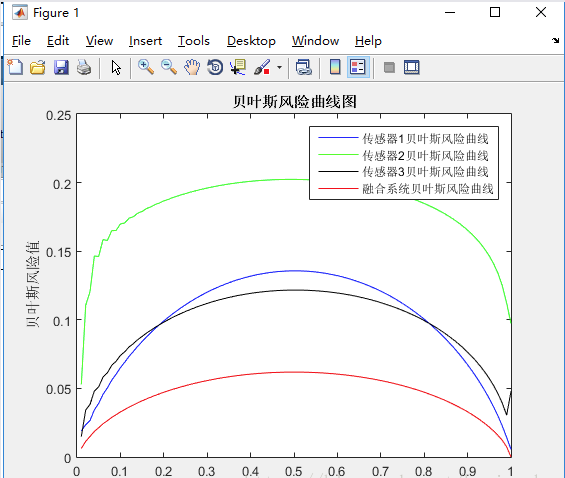https://blog.csdn.net/haxiongha/article/details/82682358